×#### Thank you for registering.

One of our academic counsellors will contact you within 1 working day.

Click to Chat

1800-1023-196

+91-120-4616500

CART 0

• 0

MY CART (5)

Use Coupon: CART20 and get 20% off on all online Study Material

ITEM
DETAILS
MRP
DISCOUNT
FINAL PRICE
Total Price: Rs.

There are no items in this cart.
Continue Shopping• Complete JEE Main/Advanced Course and Test Series
• OFFERED PRICE: Rs. 15,900
• View Details

```Chapter 24: Measures of Central Tendency Exercise – 24.2

Question: 1

Calculate the mean for the following distribution:

x:
5
6
7
8
9

f:
4
8
14
11
3

Solution:

x
f
fx

5
4
20

6
8
48

7
14
98

8
11
88

9
3
27

N = 40
∑fx = 281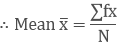= 281/40 = 7.025.

Question: 2

Find the mean of the following data:

x:
19
21
23
25
27
29
31

f:
13
15
16
18
16
15
13

Solution:

x
f
fx

19
13
247

21
15
315

23
16
368

25
18
450

27
16
432

29
15
435

31
13
403

N = 106
∑fx = 2650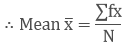= 2650/106 = 25.

Question: 3

The mean of the following data is 20.6 .Find the value of p.

x:
10
15
p
25
35

f:
3
10
25
7
5

Solution:

x
f
fx

10
3
30

15
10
150

P
25
25p

25
7
175

35
5
175

N = 50
∑fx = 25p + 530

It is given that,

Mean = 20.6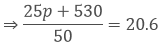⇒ 25p + 530 = 20.6 × 50

⇒ 25p = 1030 − 530

⇒ 25p = 500

⇒ p = 500/25 = 20

⇒ p = 20

∴ p = 20.

Question: 4

If the mean of the following data is 15, find p.

f:
6
p
6
10
5

x:
5
10
15
20
25

Solution:

x
f
fx

5
6
30

10
P
10p

15
6
90

20
10
200

25
5
125

N = p + 27
∑fx = 10p + 445

It is given that,

Mean = 15

⇒∑fx/N = 15

⇒10p + 445p + 27=15

⇒10p + 445 = 15 × (p + 27)

⇒ 10p + 445 = 15p + 405

⇒15p − 10p = 445 − 405

⇒ 5p = 40

⇒ p = 405 = 8

⇒ p = 8

∴ p = 8.

Question: 5

Find the value of p for the following distribution whose mean is 16.6.

x:
8
12
15
p
20
25
30

f:
12
16
20
24
16
8
4

Solution:

x
f
fx

8
12
96

12
16
192

15
20
300

P
24
24p

20
16
320

25
8
200

30
4
120

N = 100
∑fx = 24p + 1228

It is given that,

Mean = 16.6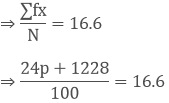⇒ 24p + 1228 = 1660

⇒ 24p = 1660 − 1228

⇒ 24p = 432

⇒ p = 432/24 = 18

⇒ p = 18

∴ p = 18.

Question: 6

Find the missing value of p for the following distribution whose mean is 12.58.

x:
5
8
10
12
p
20
25

f:
2
5
8
22
7
4
2

Solution:

x
f
fx

5
2
10

8
5
40

10
8
80

12
22
264

P
7
7p

20
4
80

25
2
50

N = 50
∑fx = 7p + 524

It is given that,

Mean = 12.58

⇒ ∑fx/N = 12.58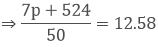⇒ 7p + 524 = 629

⇒ 7p = 629 − 524

⇒ 7p = 105

⇒ p = 1057 = 15

⇒ p = 15

∴ p = 18.

Question: 7

Find the missing frequency (p) for the following distribution whose mean is 7.68.

x
3
5
7
9
11
13

f
6
8
15
p
8
4

Solution:

x
f
fx

3
6
18

5
8
40

7
15
105

9
P
9p

11
8
88

13
4
52

N = p + 41
∑fx = 9p + 303

It is given that,

Mean = 7.68

⇒ ∑fx/N = 7.68

⇒ 9p + 303p + 41 = 7.68

⇒ 9p + 303 = 7.68p + 314.88

⇒ 9p − 7.68p = 314.88 − 303

⇒ 1.32p = 11.88

⇒ p = 11.881.32 = 9

⇒ p = 9

∴ p = 9.

Question: 8

Find the value of p, if the mean of the following distribution is 20.

x:
15
17
19
20 + p
23

f:
2
3
4
5p
6

Solution:

x
f
fx

15
2
30

17
3
51

19
4
76

20+p
5p
100p + 5p2

23
6
138

N = 5p + 15
Fx = 5p2 + 100p + 295

It is given that,

Mean = 20⇒ 5p2 + 100p + 295 = 20(5p + 15)

⇒ 5p2 + 100p + 295 = 100p + 300

⇒ 5p2 = 300 − 295

⇒ 5p2 = 5

⇒ p2 = 1

⇒ p = ±1

Frequency can’t be negative.

Hence, value of p is 1.

Question: 9

Find the mean of the following distribution:

x:
10
12
20
25
35

f:
3
10
15
7
5

Solution:

x
f
fx

10
3
30

12
10
120

20
15
300

25
7
175

35
5
175

N = 40
∑fx = 800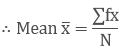= 800/40 = 20.

Question: 10

Candidates of four schools appear in a mathematics test. The data were as follows:

Schools
No. of Candidates
Average Score

I
60
75

II
48
80

III
Not Available
55

IV
40
50

If the average score of the candidates of all four schools is 66, Find the number of candidates that appeared from school III.

Solution:

Schools
No. of Candidates
Average Score

I
60
75

II
48
80

III
x
55

IV
40
50

Given the average score of all schools = 66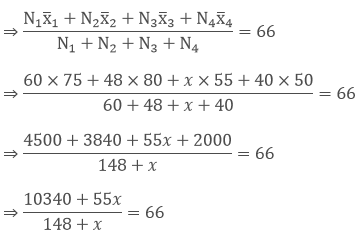⇒ 10340 + 55x = 66x + 9768

⇒ 10340 − 9768 = 66x − 55x

⇒ 11x = 572

⇒ x = 572/11 = 52

∴ No. of candidates appeared from school III = 52.

Question: 11

Five coins were simultaneously tossed 1000 times and at each, toss the number of heads was observed. The number of tosses during which 0, 1, 2, 3, 4 and 5 heads were obtained are shown in the table below. Find the mean number of heads per toss.

No. of tosses

0
38

1
144

2
342

3
287

4
164

5
25

Total
1000

Solution:

No. of heads per toss (x)
No. of tosses (f)
fx

0
38
0

1
144
144

2
342
684

3
287
861

4
164
656

5
25
125

N = 1000
∑fx = 2470

∴ Mean number of heads per toss = ∑fx/N

= 2470/1000

= 2.47

Question: 12

Find the missing frequencies in the following frequency distribution if it is known that the mean of the distribution is 50.

x:
10
30
50
70
90

f:
17
f1
32
f2
19

Solution:

x
f
fx

10
17
170

30
f1
30f1

50
32
1600

70
f2
70f2

90
19
1710

N = 120
∑fx = 3480 + 30f1 + 70f2

It is given that

Mean = 50

⇒ ∑fx/N = 50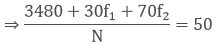⇒ 3480 + 30f1 + 70f2 = 50 × 120

⇒30f1 + 70f2 = 6000 − 3480

⇒10(3f1 + 7f2) = 10(252)

⇒ 3f1 + 7f2 = 252 ⋅⋅⋅⋅ (1)  [∵ Divide by 10]

And N = 20

⇒ 17 + f1 + 32 + f2 + 19 = 120

⇒ 68 + f1 + f2 = 120

⇒ f1 + f2 = 120 − 68

⇒ f1 + f2 = 52

Multiply with 3 on both sides

⇒ 3f1 + 3f2 = 156 ⋅⋅⋅ (2)

Subtracting equation (2) from equation (1)

⇒ 3f1 + 7f2 − 3f1 − 3f2 = 252 − 156

⇒ 4f2 = 96

⇒ f2 = 96/4 = 24

Put the value of f2 in equation (1)

⇒ 3f1 + 7 × 24 = 252

⇒ 3f1 = 252 − 168

⇒ f1 = 84/3 = 28

⇒ f1 = 28
```### Course Features

• 728 Video Lectures
• Revision Notes
• Previous Year Papers
• Mind Map
• Study Planner
• NCERT Solutions
• Discussion Forum
• Test paper with Video Solution